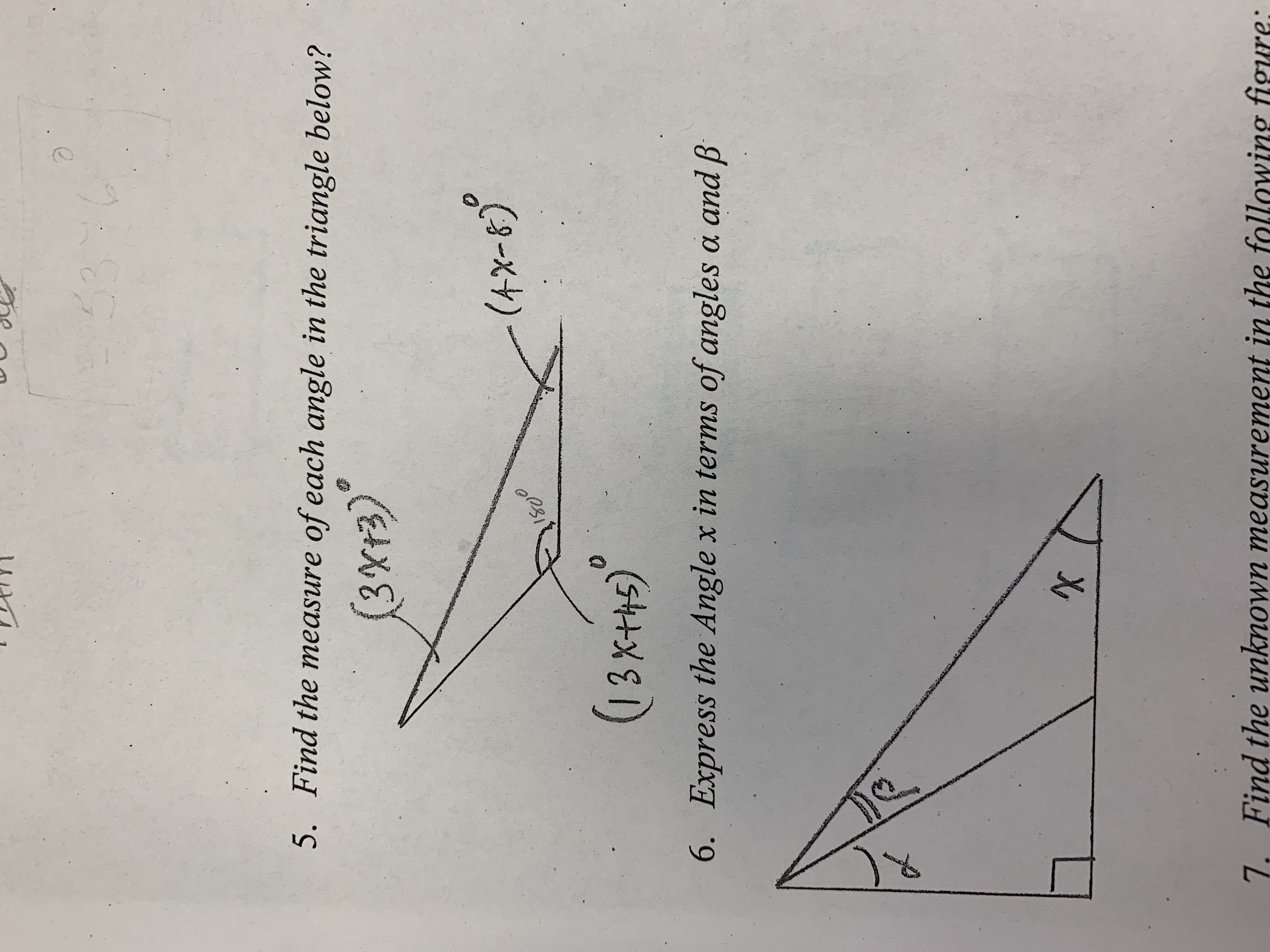# Find the measure of each angle in the triangle below? 3xt3) 0 6. Express the Angle x in terms of angles a and AB 7. Find the unknown measurement in the following figure

Question

Question 5. Find the measure of each amgle in the triangle below?

Question 6. Express the Angle x in terms of angles a and B.
Could anyone explain these questions step by step how to solve them?help_outlineImage TranscriptioncloseFind the measure of each angle in the triangle below? 3xt3) 0 6. Express the Angle x in terms of angles a and AB 7. Find the unknown measurement in the following figure fullscreen

## Expert Answer

### Want to see this answer and more?

Experts are waiting 24/7 to provide step-by-step solutions in as fast as 30 minutes!*

*Response times may vary by subject and question complexity. Median response time is 34 minutes for paid subscribers and may be longer for promotional offers.
Tagged in
Math
Trigonometry

### Other© 2021 bartleby. All Rights Reserved.